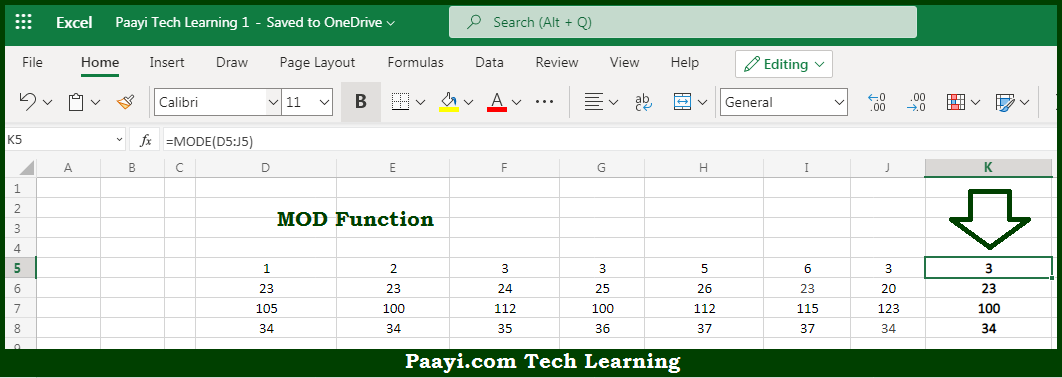# Learn How to Use Microsoft Excel MODE Function

Written by | 0 Comments | 580 Views

In this article, you will learn how to use the Microsoft Excel MODE function and its prime function in Microsoft Excel. You will also get to know the Microsoft Excel MODE function return value and syntax with the help of some examples.

Microsoft Excel MODE Function

The main purpose of the Microsoft Excel MODE function is to return the most frequently occurring number. That implies, with the help of the MODE function you can able to return the most frequently occurring number in a numeric data set provided. Let's say for example, =MODE(1,2,4,4,4,4,5,5,5,6) will return 4. So, with the help of the MODE function, you can able to get the number that has occurred most of the time in the numeric data set.

Return Value of MODE Function

The return value will be the number representing the mode.

Syntax of MODE Function

=MODE(number1, [number2], ...)

Where the arguments:

• number1: This is the number or cell reference that refers to numeric values.
• number2: This is the second number or cell reference that refers to numeric values (optional).

How to Use Microsoft Excel MODE Function?So we know that Microsoft Excel MODE function you can able to return the most frequently occurring number. That implies, with the help of the MODE function you can able to return the most frequently occurring number in a numeric data set provided. Let's say for example, =MODE(1,2,4,4,4,4,5,5,5,6) will return 4. So, with the help of the MODE function, you can able to get the number that has occurred most of the time in the numeric data set.